Courses

# Kinetic Theory Of Gases MCQ Level – 2 (part - 2)

## 10 Questions MCQ Test Topic wise Tests for IIT JAM Physics | Kinetic Theory Of Gases MCQ Level – 2 (part - 2)

Description
This mock test of Kinetic Theory Of Gases MCQ Level – 2 (part - 2) for IIT JAM helps you for every IIT JAM entrance exam. This contains 10 Multiple Choice Questions for IIT JAM Kinetic Theory Of Gases MCQ Level – 2 (part - 2) (mcq) to study with solutions a complete question bank. The solved questions answers in this Kinetic Theory Of Gases MCQ Level – 2 (part - 2) quiz give you a good mix of easy questions and tough questions. IIT JAM students definitely take this Kinetic Theory Of Gases MCQ Level – 2 (part - 2) exercise for a better result in the exam. You can find other Kinetic Theory Of Gases MCQ Level – 2 (part - 2) extra questions, long questions & short questions for IIT JAM on EduRev as well by searching above.
QUESTION: 1

### An ideal gas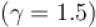is expanded adiabatically. How many times the gas to be expanded to reduce the rms velocity of molecules 2 times. Select one:

Solution:

To reduce the rms velocity two times, temperature should be reduced by 4 times.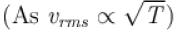∴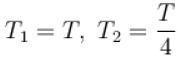V1 = V, V2 = ?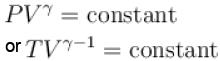⇒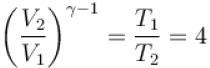⇒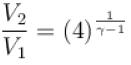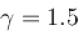⇒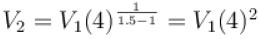⇒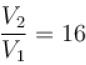The correct answer is: 16 times

QUESTION: 2

### A box containing N molecules of a perfect gas at temperature T1 and pressure p1. The number of molecules in the box is doubled keeping the total kinetic energy of the gas same as before. If the new pressure is p2 and temperature T2, then. Select one:

Solution:

Kinetic energy of N molecule of gas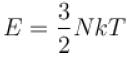Initially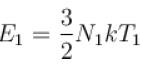and finally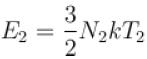But E1= E2 and N2 = 2N1
⇒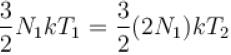⇒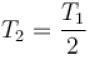Since the kinetic energy is constant
⇒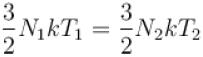⇒ N1 T1 = N2 T2
⇒ NT = constant
and  pV = NkT
⇒ p1 V1 = p2 V2
∴ p1 = p2 (as V1 = V2 )
The correct answer is: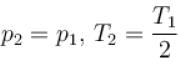QUESTION: 3

### Three closed vessels A, B and C are at the same temperature T and contain gases which obey Maxwellian distribution of velocities. Vessel A contains only O2 , B only N2 and C a mixture of equal quantities of O2 and N2. If the average speed of O2 molecules is vessel A is v1 , that of the N2 molecules in vessel R is v2 , the average speed of O2 molecule in vessel C is (where M is the mass of an oxygen molecule) Select one:

Solution:

Average speed of gas molecules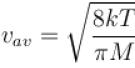It depends on temperature and molecular mass so the average speed of oxygen will be same in vessel C and A and that is equal to v1.

QUESTION: 4

Gas at pressure p0 contained in a vessel. If the masses of all the molecules are halved and their speeds  are doubled, the resulting pressure p will be equal to.
Select one:

Solution: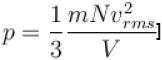⇒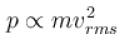⇒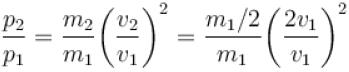⇒ p2 = 2p1 = 2p0
The correct answer is: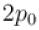QUESTION: 5

Cooking gas containers are kept in a lorry moving with uniform speed. The temperature of the gas molecule inside will.
Select one:

Solution:

If a lorry is moving with constant velocity than the vrms of gas molecules inside the container will not along and we know that T ∝ vrms so temperature remains same.
The correct answer is: Remain same

QUESTION: 6

The graph which represents the variation of mean kinetic energy of molecules with temperature t°C is.
Select one:

Solution:

Mean kinetic energy of the molecule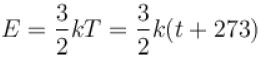where
T = temperature in Kelvin and
t = temperature in Celsius
⇒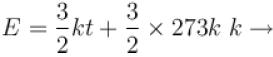Boltzmann constant
Comparing with  y = mx + c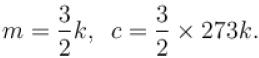⇒ Graph between E and t will be a straight line with positive intercept on E-axisand positive slope with t-axis.
The correct answer is: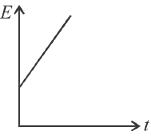QUESTION: 7

At what temperature is the root mean square velocity of gaseous hydrogen molecules is equal to that of the oxygen molecules at 47°C.
Select one:

Solution:

For oxygen O2,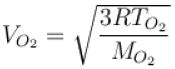and for hydrogen H2,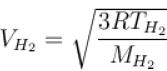According to problem,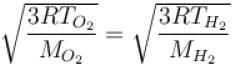⇒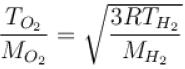⇒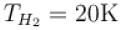The correct answer is: 20 K

QUESTION: 8

An air bubble of volume V0 is released by a fish at a depth h in a lake. The bubble rises to the surface. Assume constant temperature and standard atmosphere pressure P above the lake. The volume of the bubble just before touching the surface will be (density of water is ρ.
Select one:

Solution:

According to Boyle’s law, multiplication of pressure and volume will remain constant at bottom and top.
If p is atmospheric pressure at the top of the lake and the volume of bubble is V then
p1V1 = p2 V2
⇒ (p + hρp)V0 = pV
⇒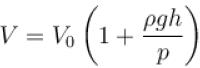The correct answer is: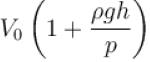QUESTION: 9

A gas is filled in the cylinder shown below. The pistons are joined by a string. If the gas is heated, the piston will.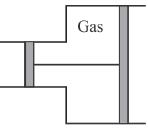Select one:

Solution:

When temperature of gas increases, it expands. As the cross-sectional area of right piston is more, therefore greater force will work on it (F = PA). So piston will move towards right.
The correct answer is: Move towards right

QUESTION: 10

The figure below shows graph of pressure and volume of a gas at two temperatures T1 and T2 which of the following inferences is correct?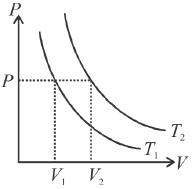Select one:

Solution:

For a given pressure, volume will be more if temperature is more (Charle’s law) From the graph, it is clear that V2 > V1
∴ T2 > T1